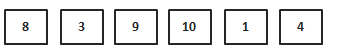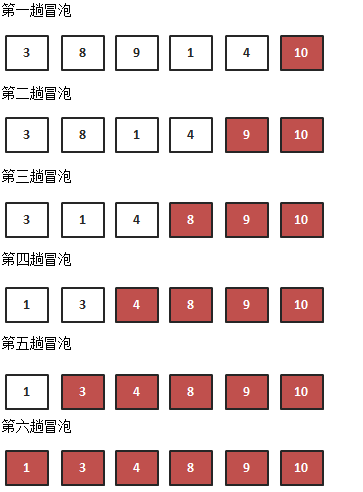# 迹忆客专注技术分享

## 排序算法学习之路——冒泡排序1）比较相邻的元素。如果第一个比第二个大，就交换他们两个。
2）对每一对相邻元素作同样的工作，从开始第一对到结尾的最后一对。这步做完后，最后的元素会是最大的数。
3）针对所有的元素重复以上的步骤，除了最后一个。
4）持续每次对越来越少的元素重复上面的步骤，直到没有任何一对数字需要比较。

``````/**
* 交换函数
*/
function swap(&\$arr,\$a,\$b){
\$t = \$arr[\$a];
\$arr[\$a] = \$arr[\$b];
\$arr[\$b] = \$t;
}
function BubbleSort(&\$arr){
\$end = count(\$arr)-1;
while(\$end>0){
for(\$i=0;\$i<\$end;\$i++){
if(\$arr[\$i]>\$arr[\$i+1]){
swap(\$arr,\$i,\$i+1);
}
}
\$end--;
}
}
\$arr = array(10,6,8,23,4,1,17,56,32,50,11,9);
BubbleSort(\$arr);
print_r(\$arr);
``````

``````function BubbleSort(&\$arr){
\$end = count(\$arr)-1;
while(\$end>0){
\$flag = false;  //每次冒泡前 初始化标志位为false
for(\$i=0;\$i<\$end;\$i++){
if(\$arr[\$i]>\$arr[\$i+1]){
swap(\$arr,\$i,\$i+1);
\$flag = true;  //有交换产生 将标志位置为true
}
}
if(!\$flag) break;  //如果没有交换产生 则直接跳出循环
\$end--;
}
}
``````## 热门标签